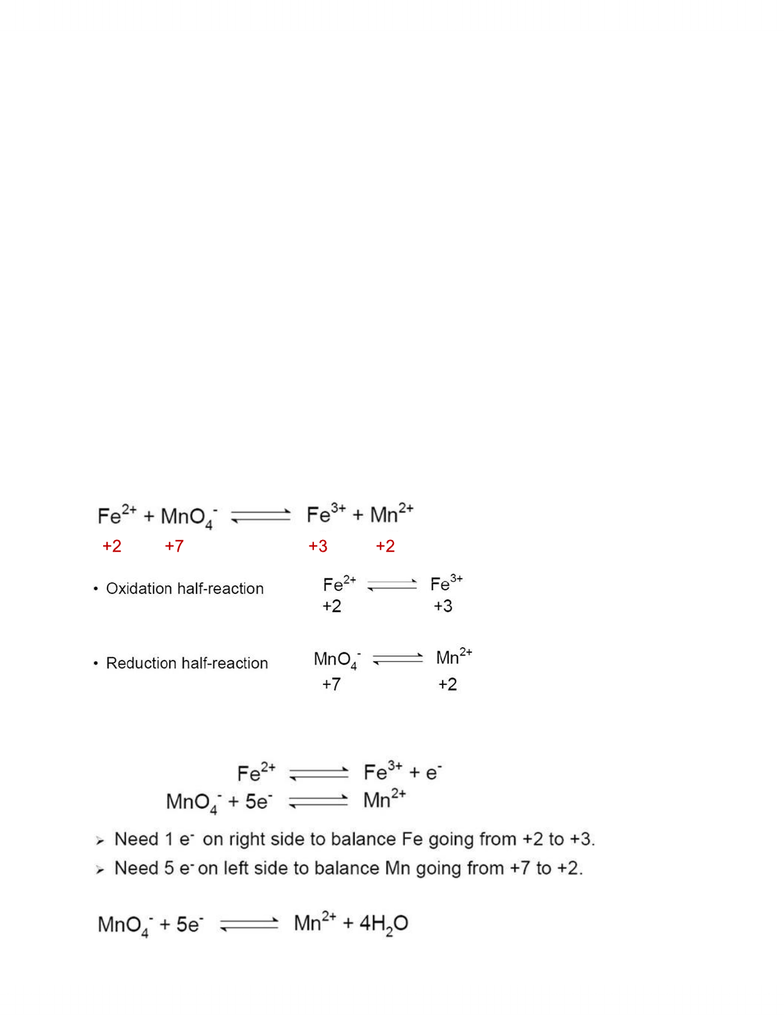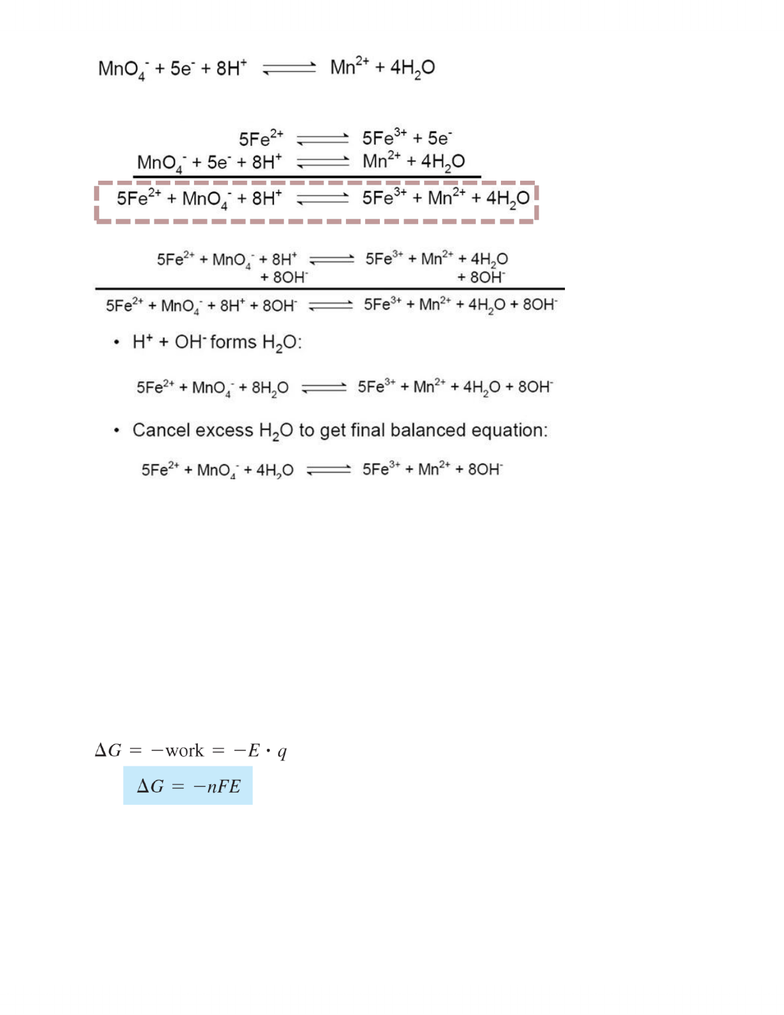# CHMB16H3 Lecture Notes - Lecture 9: Liquid Junction Potential, Standard Electrode Potential, Phase Boundary

73 views6 pages
School
UTSC
Department
Chemistry
Course
CHMB16H3
ProfessorCHMB16Fall2012 Lecture 9: Fundamentals of Electrochemistry
What is electrochemistry?
o Studies the dynamics of electrical parameters in relation to specific chemical properties
o Use of electrical measurements to draw info from a chemical system
o Use of electricity to drive a chemical reaction
o Use of a chemical reaction to produce electricity
Oxidation and Reduction Reaction (Redox reactions)
o Oxidation/reduction reactions involve the transfer of electrons and a change in oxidation state
o A reducing age (reductant) is an electron donor
o An oxidizing agent (oxidant) is an electron acceptor
Rules for Determining Oxidation Numbers
o Oxidation number of an element is itself is 0
o H is +1 unless it is in a metal hydride where H is -1
o O is -2 unless it is in peroxide where it is -1
o Alkali metals (Na, Li, K etc) are +1
o Alkaline earth metals (Be, Mg, Ca etc) are +2
o Halogens (F, Cl, Br, I) are -1
o The sum of oxidation numbers of each atom in a molecule MUST be equal to the charge of that
molecule
Balancing Redox Reactions
o Assign oxidation numbers
o
o break the reactions into its 2 half reactions
o
o balance the atoms being oxidized/reduced for each half reaction in this example the atoms are
o balance the electrons for each half reaction
o
o balance the oxygen atoms for each half reaction by adding H2O
o
o balance the hydrogen atoms for each half reaction
9
Balancing Redox Reactions
STEP 5. Balance the oxygen atoms for each half-reaction by adding H2O
STEP 6. Balance the hydrogen atoms foe each half-reaction
* Now both half-reactions should be balanced!
+2 +7 +3 +2
Unlock document

This preview shows pages 1-2 of the document.
Unlock all 6 pages and 3 million more documents.o
o multiply to balance and add the half reactions together to get the net equation this example is
balancing in acidic conditions
o
o if you have to balance in basic conditions, add OH- equal to H+ on both sides of the reaction
o
Charge
o q=nF where F = 9.649 x 104 C/mol e-
o studies the dynamics of electrical parameters (current and potential) in relation to specific
chemical properties
o electric current: the amount of charge flowing past a point in a circuit each second measured in
A (1A = 1C/s)
o electrical work: the electric potential (E) between 2 points is the work needed when moving
charge between 2 points
o this potential is measured in V
o when moving a charge q through a potential difference E the work done is:
o W =Eq
Free Energy Change
o
Electrochemical Cells
o Galvanic cell : does work, provides E through spontaneous electrochemical reaction
o Electrolytic cell: requires work, E must be externally applied to drive the chemical reaction
o Both types of cells can be also divided into reversible and irreversible cells
o Reversible: reversing the direction of electron flow causes the direction of the electrochemical
reactions to reverse
9
Balancing Redox Reactions
STEP 5. Balance the oxygen atoms for each half-reaction by adding H2O
STEP 6. Balance the hydrogen atoms foe each half-reaction
* Now both half-reactions should be balanced!
+2 +7 +3 +2
10
Balancing Redox Reactions
STEP 7. Multiply and add each half reaction together to get the net equation
+2 +7 +3 +2
Balanced!
11
Unlock document

This preview shows pages 1-2 of the document.
Unlock all 6 pages and 3 million more documents.

## Document Summary

Oxidation and reduction reaction (redox reactions: oxidation/reduction reactions involve the transfer of electrons and a change in oxidation state, a reducing age (reductant) is an electron donor, an oxidizing agent (oxidant) is an electron acceptor. Balance the oxygen atoms for each half-reaction by adding h2o step 6. Balance the hydrogen atoms foe each half-reaction *now both half-reactions should be balanced! +2 +7 +3 +2 10 balancing redox reactions step 7. Multiply and add each half reaction together to get the net equation +2 +7 +3 +2 balanced! K+ ions flowing out of the salt bridge at the cathode. Redox reactions in an electrochemical cell: ecell can be measured using a voltmeter connected between the 2 electrodes of the cell. 23 electrode potentials e - anode cathode e - o as a result, the measured potential is not an absolute half cell potential but instead the difference between the 2 absolute half cell potentials.